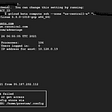# Level Up Your Python Programming With Itertools

## An introduction to itertools module in Python to level up programming

Python's real power lies in using its own modules to compose its functions to create fast, memory-efficient, and good-looking code. We will look at some examples of the itertools to understand its applications.

According to Python official docs “Functions creating iterators for efficient looping”. itertools provides us with three different types of iterators

• Terminating iterators
• Infinite iterators
• Combinatoric iterators

# Terminating iterators

These iterators are used to work on finite sequences and produce an output based on the function used.

## accumulate()

`itertools.accumulate(iterable[, func])`

This function makes an iterator that returns the results of a function.

`data = [1, 2, 3, 4, 5]result = itertools.accumulate(data, operator.mul)for each in result:    print(each)`

Output

`12624120`

As the 2nd parameter (function) is optional by default accumulate would sum list elements.

`data = [1, 2, 3, 4, 5]result = itertools.accumulate(data)for each in result:    print(each)`

Output

`1361015`

## chain()

`itertools.chain(*iterables)`

This function takes a series of iterables and returns them as one long iterable.

`for each in itertools.chain(['A', 'B', 'C'], ['D', 'E', 'F']):    print(each)`

Output

`ABCDEF`

It has a function `chain.from_iterabe()` which works in a similar fashion, but it takes an argument as a list of lists instead of multiple lists.

`for each in itertools.from_iterable([['A', 'B', 'C'], ['D', 'E', 'F']]):    print(each)`

Output

`ABCDEF`

## compress()

`itertools.compress(data, selectors)`

This function filters one iterable with another.

`countries = ['Pakistan', 'China', 'India', 'Afghanistan']selections = [True, False, True, False]result = itertools.compress(countries, selections)for each in result:    print(each)`

Output

`PakistanIndia`

## dropwhile()

`itertools.dropwhile(predicate, iterable)`

Make an iterator that drops elements from the iterable as long as the predicate is true; afterward, returns every element.

`data = [1, 2, 3, 4, 5, 6, 7, 8, 9, 10]result = itertools.dropwhile(lambda x: x<5, data)for each in result:    print(each)`

Output

`5678910`

# Infinite iterators

These iterators do not exhaust, unlike lists and dictionaries.

## count()

`itertools.count(start=0, step=1)`

Makes an iterator that returns evenly spaced values starting with number start.

`for i in itertools.count(1,3):    print(i)    if i > 10:        break`

In the above code, we iterate or loop over a function. We tell the function to start at 1 and take a step of 3.

Output

`1369`

## cycle()

`itertools.cycle(iterable)`

This function cycles through an iterator endlessly.

`numbers = [1, 2, 3]for number in itertools.cycle(numbers):    print(number)`

In the above code, we create a list. Then we cycle or loop through this list endlessly. Normally, a `for` loop steps through an iterable until it reached the end. If a list has 3 items, the loop will iterate 3 times. If we use the `cycle()` function it wouldn’t stop after 3 times. With this function, when we reach the end of the iterable we start over again from the beginning.

Output

`123123123123123123....`

Note: The endless output above is truncated with ellipses.

# Combinatoric iterators

## combinations()

`itertools.combinations(iterable, r)`

This function takes an iterable and an integer. This will create all the unique combination that has `n` members.

`numbers = [1, 2, 3]result = itertools.combinations(numbers, 2)for each in result:    print(each)`

In this code, we make all combos with 2 members.

Output

`(1, 2)(1, 3)(2, 3)`

## permutations()

`itertools.permutations(iterable, r=None)`

Return successive `n` length permutations of elements in the iterable.

`alphabets = ['a', 'b', 'c']result = itertools.permutations(alphabets)for each in result:    print(each)`

Output

`('a', 'b', 'c')('a', 'c', 'b')('b', 'a', 'c')('b', 'c', 'a')('c', 'a', 'b')('c', 'b', 'a')`

--

--

--

## More from Geek Culture

A new tech publication by Start it up (https://medium.com/swlh).

## GCP: Resize/Repair Compute engine root partition is full (100%) issues on the fly.## Best Practices for Salesforce CPQ Implementation## AWS System Manager — Manage Server remotely## SINOVATE Weekly Update 19/04/2021 ~ 26/04/2021A passionate engineer with experience in architecting, building, and maintaining scalable softwares. Connect with me: https://twitter.com/haseeb__tweets

## 7 Difficult Python Test Questions That Many Could Not Solve In Time## How Do You Get Unique Characters in a String? Python Basics Explained## Python 101: Introduction to Modern Python## A journey from easy to robust python code